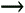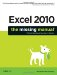# Section 19.2. Using Goal Seek

19.2. Using Goal Seek

There are some problems that formulas just can't crack. One of these is solving questions that need trial-and-error guesswork.

In most Excel spreadsheets, you begin with a set of data and use formulas to analyze that data and calculate some conclusions. When you create plans and projections, however, you sometimes invert this process and start with the conclusions. For example, you might begin with a profit projection and calculate "backward" to find out the sales you need to make. Or, you might calculate the rate an investment needs to increase in value in order to meet a set target.

Goal seeking is a fairly simple tool in Excel that can help you answer these sorts of questions, provided your problem meets the following guidelines:

• There's only one result cell and this cell contains a formula . You want to make sure the result of this calculation meets a target you specify.

• There's only one variable cell . This is the value that Excel adjusts to meet your target in the result cell.

• There's a valid solution . For example, if you ask Excel how many years it'll take to pay off a \$100,000 loan by making 25-cent monthly payments, you'll end up with an answera negative number of yearsthat makes no sense (since your loan is accumulating interest faster than you're making payments).

To better understand goal seeking, it helps to think about a simple example. Consider the worksheet shown in Figure 19-7, which shows a list of student grades. Grace Dewitt is given the chance to resubmit the assignment, and she wants to determine what grade she needs on the assignment to increase her final grade from 72 to 80 out of a possible 100.Figure 19-7. Grace Dewitt is trying to determine the grade she needs on an assignment to achieve a final grade of 80 percent (which is the target). In this case, the result cell (the one she wants to optimize) is E3. The variable cell (the one that Excel will adjust) is D3.

To use goal seeking in this scenario, just follow these steps:

1. Choose ToolsGoal Seek.

The Goal Seek dialog box appears (Figure 19-8).Figure 19-8. This example shows the Goal Seek dialog box filled with the values needed to search for the minimum grade Grace needs to get on the assignment to increase her final grade to 80. Note that you have to enter the grade as a decimal value (0.80 instead of 80). That's because the cells in column E have been formatted using the percentage number style (as explained on Section 4.1.1.5).

2. In the "Set cell" text box, enter the cell you want Excel to optimize.

In the student grade example, this is cell E3, which contains Grace's final grade.

3. In the "To value" text box, enter your target.

Grace is seeking a final grade of 80 percent, so the target is 0.80 .

4. In the "By changing cell" text box, enter the cell that Excel should modify to achieve the target.

Cell D3, which contains the assignment grade, is the value that Excel modifies to determine what grade she needs to get on the assignment in order to boost her final grade to 80.

5. Click OK to start goal seeking.

The Goal Seek Status dialog box appears, and Excel cycles through a series of trial values, incrementing the assignment grade and calculating the resulting value.

If Excel can't find the answer after a series of attempts, the Goal Seek Status dialog box gives you the option to keep trying by clicking Step. If Excel stalls on a long analysis, you can stop it by clicking Pause, although this move is rarely necessary. In most cases, the goal-seeking process finishes before you can even take your hand off the mouse to reach for a nearby coffee mug.

In a short amount of time, Excel discovers that an assignment grade of 91 (out of 100) raises the final grade to 80 percent. At this point, it stops the goal seeking process and shows you the answer.

Figure 19-9 shows the adjusted worksheet.

#### 19.2.1. Goal Seeking with Complex Equations

In the student grade example, you don't necessarily need to use goal seeking to get the answer you're looking for. You could get the same information by rewriting the equation. To understand how this approach works, it helps to take a closer look at the formula that calculates the final grade:

` =(B3/B12)*25% + (C3/C12)*25% + (D3/D12)*50% `

In this formula, the two tests are each worth 25 percent of the final grade, and the assignment is worth the remaining 50 percent. However, using a dash of high school math, you can rearrange this formula to find what grade you need on the assignment to get an 80 percent overall grade ( assuming you already know your grades on the two tests). Here's the answer:

` =(80% - (B3/B12)*25% - (C3/C12)*25%)/50%*D12 `

This formula looks a little intimidating at first glance, but it's really not too difficult to understand. First of all, you start with the 80% final grade you're trying to achieve. You subtract the two test scores to find the value you need from the assignment. Finally, you divide that value by 50 percent (the weighting of the assignment) and multiply it by D12 (the total score available in the assignment). The end result is 91.25.Figure 19-9. Usually, goal seeking unfolds in a flash. In this example, Excel has met the target by setting the assignment grade to 91, as shown in cell D3. You can click OK to accept the change permanently, or Cancel to return the cell to its previous value.

Based on this example, you might assume that goal seeking is just a tool for quickly calculating a number that you could obtain on your own by rewriting one or more formulas. Although that's often the case, there are some types of formulas that you can't reorganize to provide an answer. These formulas often have exponents and use the value in the variable cell more than once. You can find many examples of this sort of equation in the scientific world.

One common example is the quadratic function , which shows up in lots of different scientific modeling and engineering formulas. A typical quadratic formula looks like this:

` y = x  2  + 5x + 10 `

In this case, it's easy to calculate the result (y) provided you know the input value (x). However, it's much more difficult to perform the reverse taskdetermining x if all you know is y. Try as you might, there's no way to rearrange the formula to solve the problem. In this case, goal seeking becomes very useful. It uses a trial-and-error approach that can usually get the answer you need. Excel tries different values of x in succession trying to get closer and closer to the target result.

To put the problem into Excel's formula format, assume that cell B1 contains the x value. Here's what the formula would look like:

` =B1^2 + 5*B1 + 10 `

Figure 19-10 shows an example worksheet that puts this function to the test. Clearly, it's easy to see that an input value of 20 produces a result of 510. But what input value do you need to get a result of 1000? In this example, goal seeking is the only easy way to find the answer (which, incidentally, is 29.0643).Figure 19-10. Top : Figuring out what the value of B1 needs to be to generate a formula result of 1,000 is extremely difficult to resolve without using goal seeking. Bottom : As you can see in the Goal Seek Status dialog box, the answer isn't perfect (it's 1000.000002 instead of 1000), but it's close enough for Excel to stop searching. When performing goal seeking, Excel doesn't need to match your target exactly.

Note: Another case where it's often difficult to reverse engineer a formula is in financial calculations, like those that calculate how an investment appreciates in value. However, as you saw in Chapter 9, Excel includes financial functions that can calculate in both directions: these functions can calculate the final value of an investment based on a given interest rate, or calculate the required interest rate or investment length based on your desired goal. Of course, you can still use goal seeking with financial functions to find an answer quickly without writing a new formula.Excel 2010: The Missing Manual
ISBN: 1449382355
EAN: 2147483647
Year: 2003
Pages: 185